Test: Fluid Mechanics Level - 4

# Test: Fluid Mechanics Level - 4

Test Description

## 25 Questions MCQ Test Civil Engineering SSC JE (Technical) | Test: Fluid Mechanics Level - 4

Test: Fluid Mechanics Level - 4 for Civil Engineering (CE) 2022 is part of Civil Engineering SSC JE (Technical) preparation. The Test: Fluid Mechanics Level - 4 questions and answers have been prepared according to the Civil Engineering (CE) exam syllabus.The Test: Fluid Mechanics Level - 4 MCQs are made for Civil Engineering (CE) 2022 Exam. Find important definitions, questions, notes, meanings, examples, exercises, MCQs and online tests for Test: Fluid Mechanics Level - 4 below.
Solutions of Test: Fluid Mechanics Level - 4 questions in English are available as part of our Civil Engineering SSC JE (Technical) for Civil Engineering (CE) & Test: Fluid Mechanics Level - 4 solutions in Hindi for Civil Engineering SSC JE (Technical) course. Download more important topics, notes, lectures and mock test series for Civil Engineering (CE) Exam by signing up for free. Attempt Test: Fluid Mechanics Level - 4 | 25 questions in 50 minutes | Mock test for Civil Engineering (CE) preparation | Free important questions MCQ to study Civil Engineering SSC JE (Technical) for Civil Engineering (CE) Exam | Download free PDF with solutions
 1 Crore+ students have signed up on EduRev. Have you?
Test: Fluid Mechanics Level - 4 - Question 1

### When a block of ice floating on water in a container melts, the level of water in the container

Test: Fluid Mechanics Level - 4 - Question 2

### When a ship enters sea from a river one can expect it

Test: Fluid Mechanics Level - 4 - Question 3

### A large metacentric height in a vessel

Test: Fluid Mechanics Level - 4 - Question 4

The time of oscillation of a floating body is given by
Where
K = Radius of Gyration of the floating body about its centre of gravity
h = Metacentric height of the floating body

Test: Fluid Mechanics Level - 4 - Question 5

A cylindrical body of cross-sectional area A height H and density ρs is immersed to a depth h in a liquid of density ρ and tied down to bottom with a string then the tension in the string is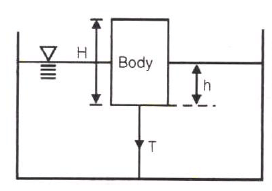Test: Fluid Mechanics Level - 4 - Question 6

There is no geometrical distinction between the streamline, pathline and streakline in case of

Test: Fluid Mechanics Level - 4 - Question 7

Stream line, path line and streak line are identical when the

Test: Fluid Mechanics Level - 4 - Question 8

Match List I with List II and select the correct answer using the codes given below the lists: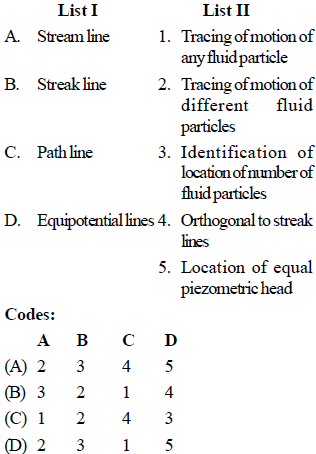Test: Fluid Mechanics Level - 4 - Question 9

The continuity equation in differential form is

Test: Fluid Mechanics Level - 4 - Question 10

Under which of the following conditions will the equation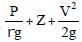= constant, will be valid in the whole flow field ?

1. Flow is rotational

2. Flow is irrotational

3. Flow is incompressible

5. Flow is laminar

Test: Fluid Mechanics Level - 4 - Question 11

​Venturimeter consists of following parts :

1. Diverging part

2. Throat

3. Main inlet pipe

4. Short converging part

Select the correct sequence of parts:

Test: Fluid Mechanics Level - 4 - Question 12

The range for coefficient of discharge (Cd) for a venturimeter is

Test: Fluid Mechanics Level - 4 - Question 13

In the siphon in figure below assuming ideal flow, the pressure PB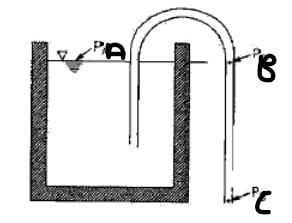Detailed Solution for Test: Fluid Mechanics Level - 4 - Question 13

As PA = PC both have atmospheric pressure and keeping other factors of energy constant, as liquid is flowing from B to C, PB<PC
Hence PB<PA

Test: Fluid Mechanics Level - 4 - Question 14

The flow of fluid through a pipe is laminar when

Test: Fluid Mechanics Level - 4 - Question 15

An oil of kinematic viscosity 0.5 stokes flows through a pipe of 4 cm diameter. The flow is critical at a velocity of about

Test: Fluid Mechanics Level - 4 - Question 16

In the Navier-Stokes equations, the forces considered are

Test: Fluid Mechanics Level - 4 - Question 17

Navier-Stoke's equation represents the conservation of

Detailed Solution for Test: Fluid Mechanics Level - 4 - Question 17

The Navier-Stokes equations represent the conservation of momentum, while the continuity equation represents the conservation of mass.

Test: Fluid Mechanics Level - 4 - Question 18

For laminar flow between two fixed parallel plates, the flow velocity

Detailed Solution for Test: Fluid Mechanics Level - 4 - Question 18

Explanation : Laminar flow, type of fluid (gas or liquid) flow in which the fluid travels smoothly or in regular paths, in contrast to turbulent flow, in which the fluid undergoes irregular fluctuations and mixing. ... The fluid in contact with the horizontal surface is stationary, but all the other layers slide over each other.

The velocity profile is a parabola.

Test: Fluid Mechanics Level - 4 - Question 19

Capillary tube viscometers used for measurement of viscosity are based on

Test: Fluid Mechanics Level - 4 - Question 20

The kinematic viscosity of oil of specific gravity .8 is .0005 .This oil is used for lubrication of shaft of diameter .4 m and rotates at 190 rpm. Calculate the power lost in the bearing for a sleeve length of 90mm. The thickness of the oil film is 1.5mm.

Detailed Solution for Test: Fluid Mechanics Level - 4 - Question 20

Power lost= torque * angular velocity
= shear stress * area* radius* angular velocity
Shear Stress = viscosity* velocity gradient
Power lost= 0.0005*0.8*1000* 2*3.142*190/60*0.2*3.142*0.23 * 190/60
= 477.65 Watts.

Test: Fluid Mechanics Level - 4 - Question 21

Pressure drop in a 100 mm diameter horizontal pipe is 50 kPa over a length of 10 m. The shear stress at the pipe wall is

Detailed Solution for Test: Fluid Mechanics Level - 4 - Question 21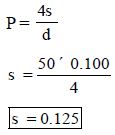Test: Fluid Mechanics Level - 4 - Question 22

The pressure drop for a relatively low Reynolds number flow in a 600 mm, 30 m long pipe line is 70 kPa. What is the wall shear stress?

Detailed Solution for Test: Fluid Mechanics Level - 4 - Question 22

Use,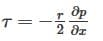Test: Fluid Mechanics Level - 4 - Question 23

The body whose surface does not coincide with the streamline when places in a flow is called as

Test: Fluid Mechanics Level - 4 - Question 24

Shear velocity is

Test: Fluid Mechanics Level - 4 - Question 25

The head loss in a sudden expansion from 4 cm diameter pipe to 6 cm diameter pipe in terms of velocity V1 in the smaller diameter pipe is:

## Civil Engineering SSC JE (Technical)

2 videos|111 docs|50 tests
 Use Code STAYHOME200 and get INR 200 additional OFF Use Coupon Code
Information about Test: Fluid Mechanics Level - 4 Page
In this test you can find the Exam questions for Test: Fluid Mechanics Level - 4 solved & explained in the simplest way possible. Besides giving Questions and answers for Test: Fluid Mechanics Level - 4, EduRev gives you an ample number of Online tests for practice

## Civil Engineering SSC JE (Technical)

2 videos|111 docs|50 tests